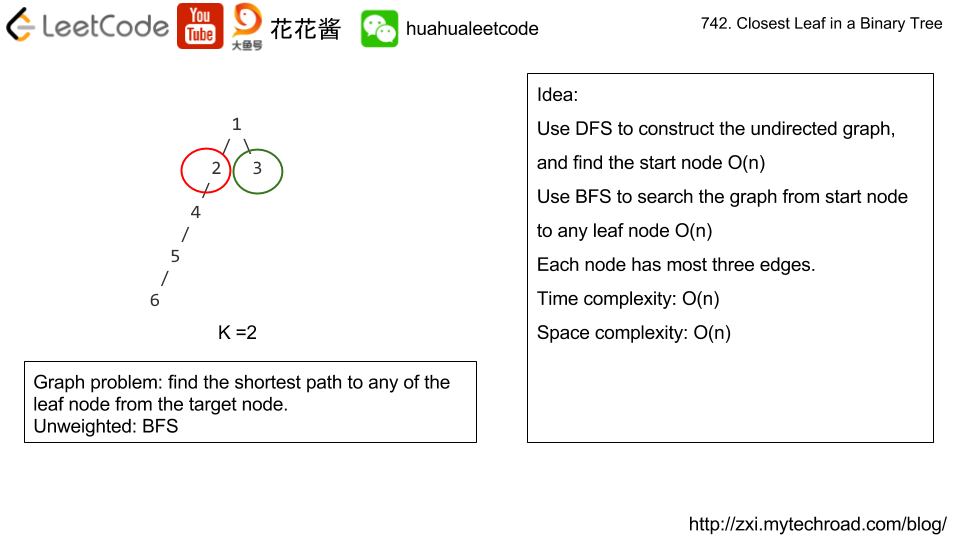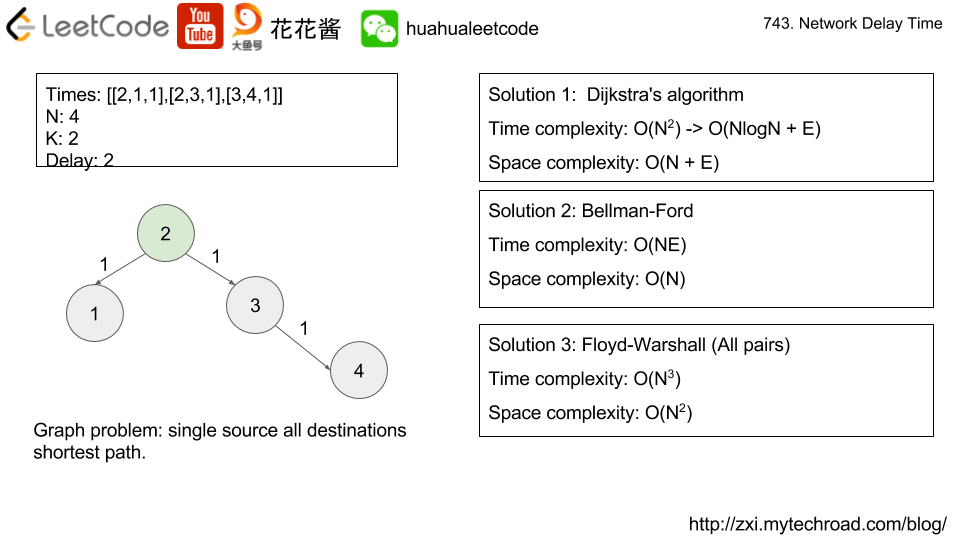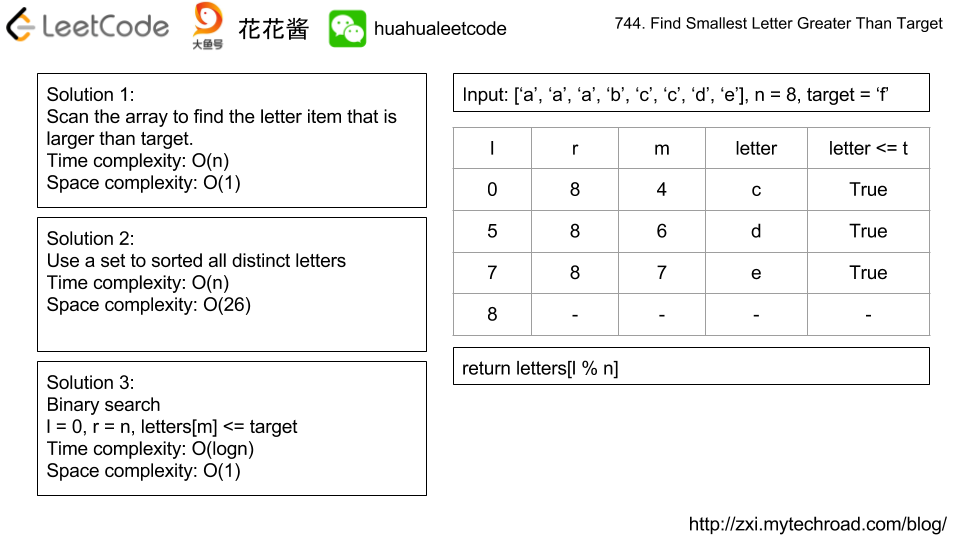Problem:

Given a binary tree where every node has a unique value, and a target key k, find the value of the closest leaf node to target k in the tree.

Here, closest to a leaf means the least number of edges travelled on the binary tree to reach any leaf of the tree. Also, a node is called a leaf if it has no children.

In the following examples, the input tree is represented in flattened form row by row. The actual root tree given will be a TreeNode object.

Example 1:

Example 2:

Example 3:

Note:

1. root represents a binary tree with at least 1 node and at most 1000 nodes.
2. Every node has a unique node.val in range [1, 1000].
3. There exists some node in the given binary tree for which node.val == k.

Idea:

Shortest path from source to any leaf nodes in a undirected unweighted graph.Solution:

C++ / DFS + BFS

Time complexity: O(n)

Space complexity: O(n)

There are N network nodes, labelled 1 to N.

Given times, a list of travel times as directed edges times[i] = (u, v, w), where u is the source node, v is the target node, and w is the time it takes for a signal to travel from source to target.

Now, we send a signal from a certain node K. How long will it take for all nodes to receive the signal? If it is impossible, return -1.

Note:

1. N will be in the range [1, 100].
2. K will be in the range [1, N].
3. The length of times will be in the range [1, 6000].
4. All edges times[i] = (u, v, w) will have 1 <= u, v <= N and 1 <= w <= 100.

Idea:

Construct the graph and do a shortest path from K to all other nodes.Solution 2:

C++ / Bellman-Ford

Time complexity: O(ne)

Space complexity: O(n)

Solution3:

C++ / Floyd-Warshall

Time complexity: O(n^3)

Space complexity: O(n^2)

v2

Problem:

Given many wordswords[i] has weight i.

Design a class WordFilter that supports one function, WordFilter.f(String prefix, String suffix). It will return the word with given prefix and suffix with maximum weight. If no word exists, return -1.

Examples:

Note:

1. words has length in range [1, 15000].
2. For each test case, up to words.length queries WordFilter.f may be made.
3. words[i] has length in range [1, 10].
4. prefix, suffix have lengths in range [0, 10].
5. words[i] and prefix, suffix queries consist of lowercase letters only.

Idea:

Construct all possible filters

Solution1:

C++

Time complexity: O(NL^3 + QL)  where N is the number of words, L is the max length of the word, Q is the number of queries.

Space complexity: O(NL^3)

Version #2

Solution 2:

C++ / Trie

Time complexity: O(NL^2 + QL)  where N is the number of words, L is the max length of the word, Q is the number of queries.

Space complexity: O(NL^2)

Related Problems:

Problem:

Given a list of sorted characters letters containing only lowercase letters, and given a target letter target, find the smallest element in the list that is larger than the given target.

Letters also wrap around. For example, if the target is target = 'z' and letters = ['a', 'b'], the answer is 'a'.

Examples:

Note:

1. letters has a length in range [2, 10000].
2. letters consists of lowercase letters, and contains at least 2 unique letters.
3. target is a lowercase letter.

Idea:

1. Scan the array Time complexity: O(n)
2. Binary search Time complexity: O(logn)Solution 1:

C++ / Scan

C++ / Set

C++ / Binary Search

https://leetcode.com/problems/maximum-gap/description/

Problem:

Given an unsorted array, find the maximum difference between the successive elements in its sorted form.

Try to solve it in linear time/space.

Return 0 if the array contains less than 2 elements.

You may assume all elements in the array are non-negative integers and fit in the 32-bit signed integer range.

Example:

Input:  [5, 0, 4, 2, 12, 10]

Output: 5

Explanation:

Sorted: [0, 2, 4, 5, 10, 12]

max gap is 10 – 5 = 5

Idea:

Bucket sort. Use n buckets to store all the numbers. For each bucket, only track the min / max value.

max gap must come from two “adjacent” buckets, b[i], b[j], j > i, b[i+1] … b[j – 1] must be empty.

max gap 只可能来自”相邻”的两个桶 b[i] 和 b[j], j > i, b[i] 和 b[j] 之间的桶（如果有）必须为空。

max gap = b[j].min – b[i].min

Time complexity: O(n)

Space complexity: O(n)

Solution:

C++

Mission News Theme by Compete Themes.